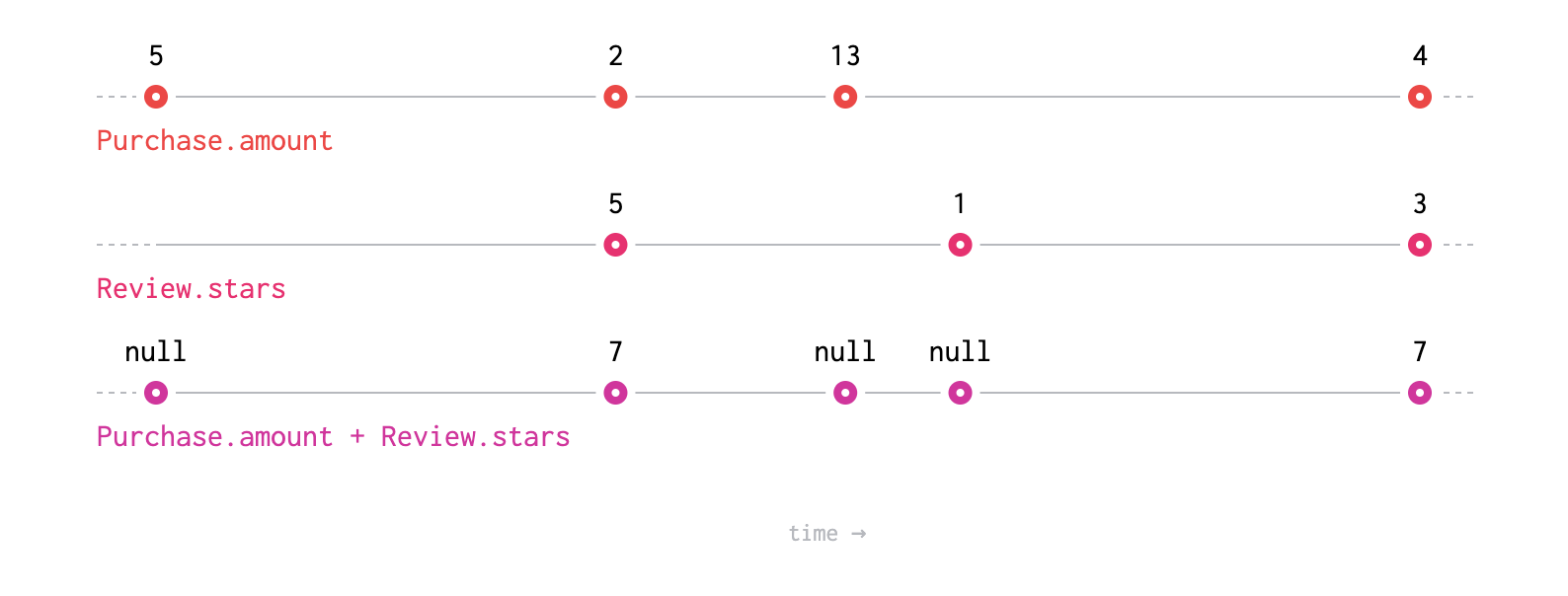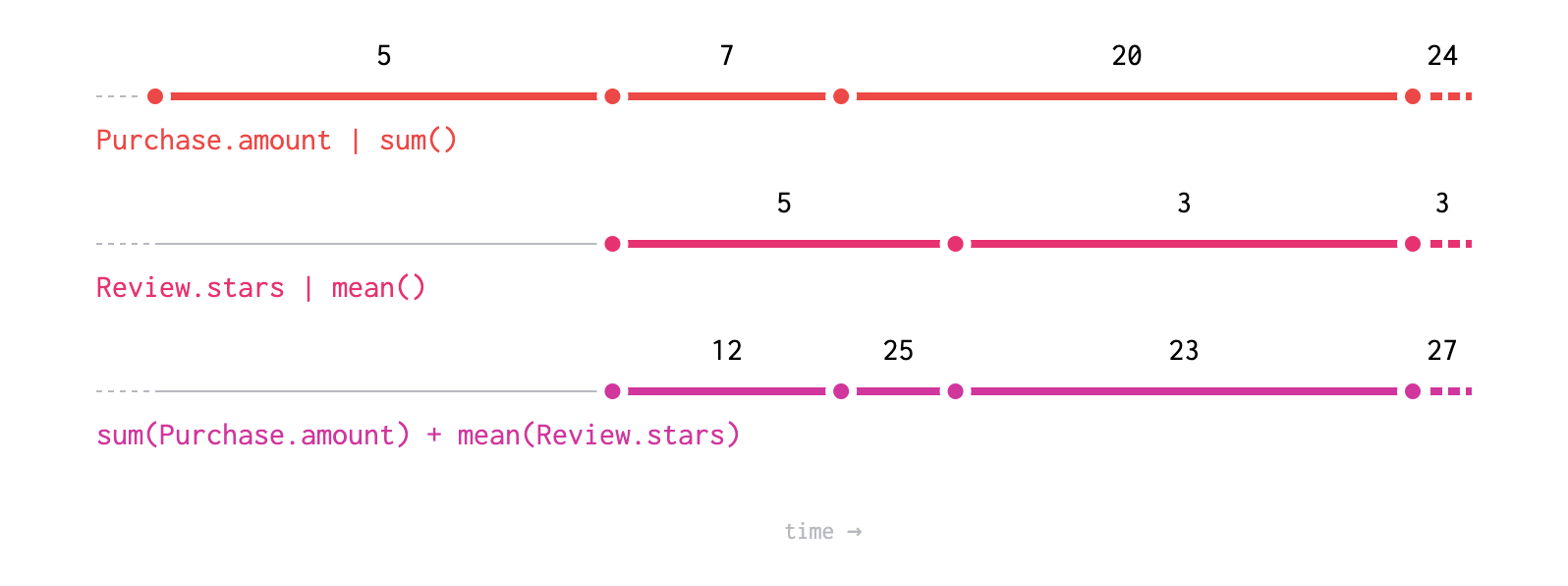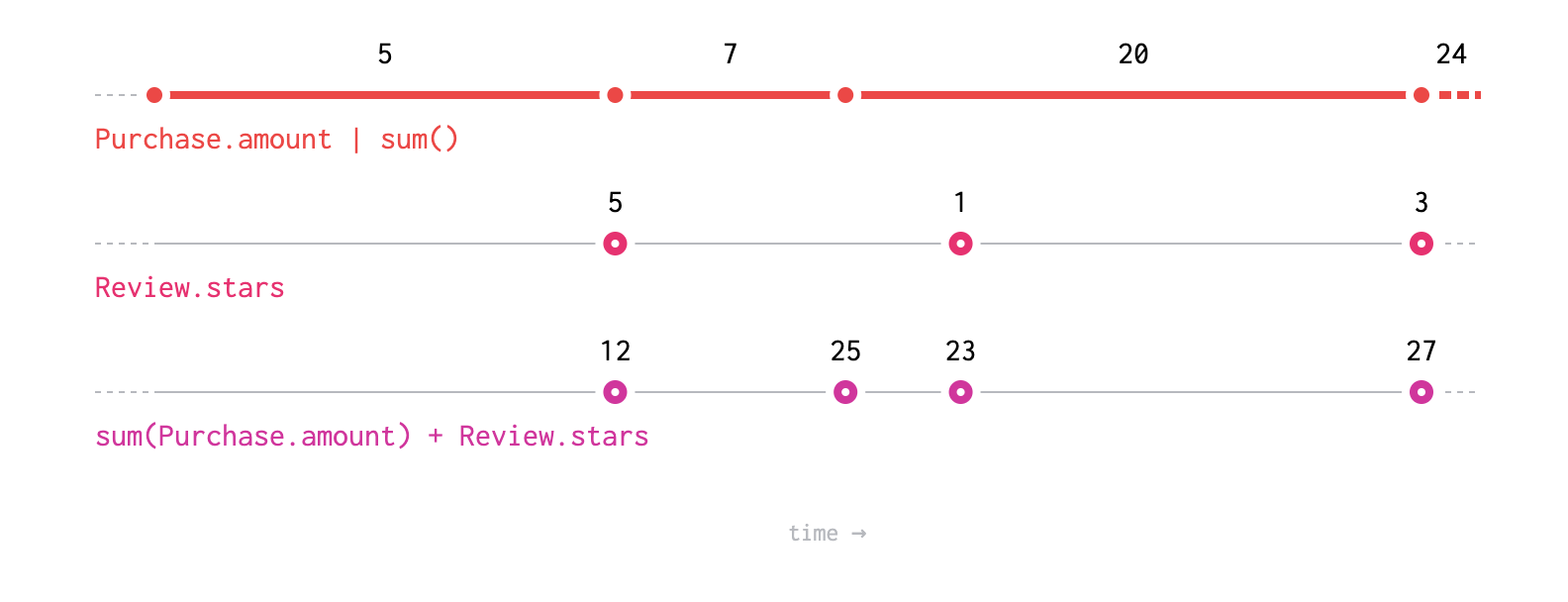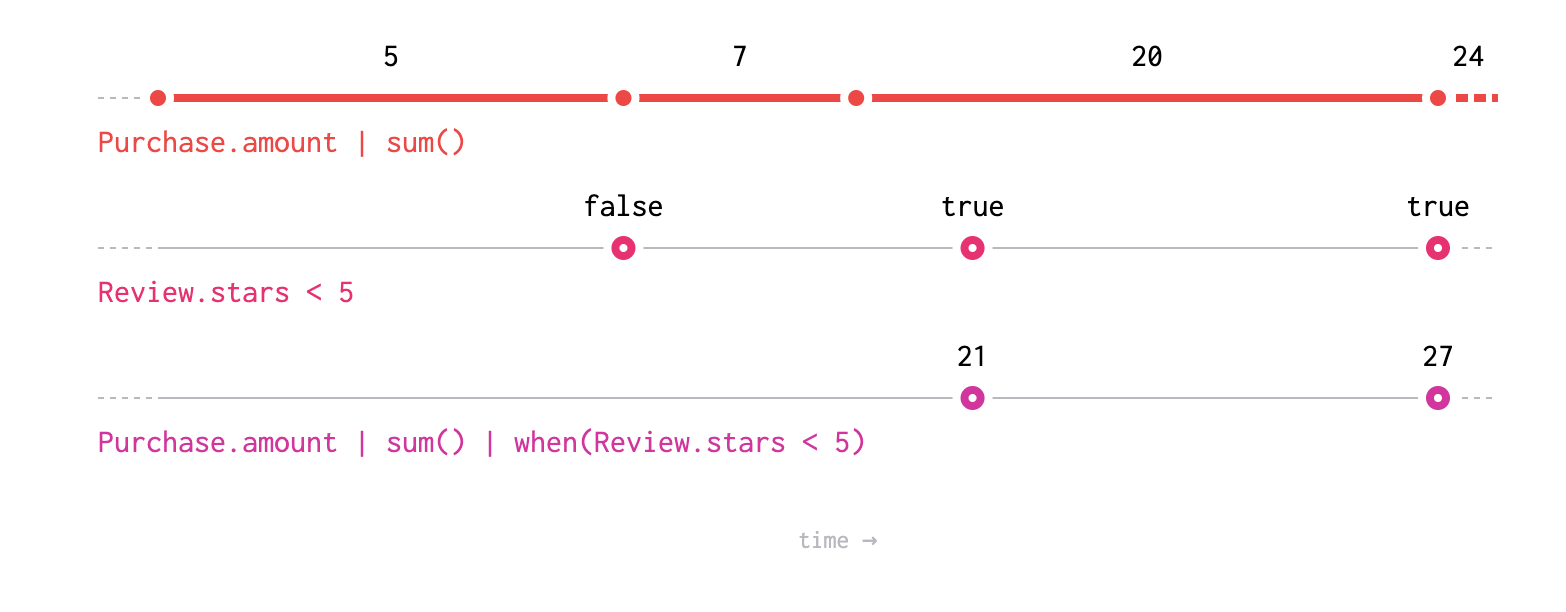# Continuity

The difference between continuous and point-in-time expressions

Fenl expressions are either discrete or continuous. Discrete expressions describe values that are only meaningful at specific times. Continuous expressions describe values that are meaningful over ranges of time.

## Discrete Expressions

A discrete expression produces values at a finite set of times, at all other times its value is `null`. Tables contain a finite set of events, and each event is associated with a specific time. A table only has a a meaningful value at the set of times for which events exist. Similarly, simple operations such as field reference produce discrete results when applied to discrete expressions.Discrete expressions may be combined in various ways, for example by adding them together as shown.

In most cases operations produce output values any time one of their input expressions produces a value. In this example we can see that many of the values produced are `null`, since the expression `Purchase.amount` is defined at different times than `Review.stars`, and adding `null` to a number produces `null`.

## Continuous Expressions

A continuous expression has a meaningful value at all points in time. Continuous expressions produce values at every time contributing to their value. Aggregations generally produce continuous values.

For example, at any point in time we can describe the sum of all `Purchase.amount` values seen so far.Continuous expressions have a value at all times, but they produce values at specific times. These times are illustrated above as circles. A continuous expression can be thought of as producing a stream of updates; at any given time the stream takes the value of the most recent update.

When continuous values are aggregated, the aggregation is updated when a value is produced. For example, `count` produces the same result for a discrete expression with and without an intermediate aggregation.

``````Purchase.amount | count() == Purchase.amount | sum() | count()
``````

### ℹ️ Design Note

The distinction between "having" a value and "producing" a value allows aggregations to be applied in the same way to both continuous and discrete expression. Applying an operation such as `sum` to a continuous value (in the mathematical sense) would correspond to integrating the value.

We feel interpreting aggregations of continuous values as integration would be surprising, especially in cases such as `count`.

## Interactions Between Continuous and Discrete Expressions

The continuity of the result of an operation involving multiple expressions depends on the continuity of the operation's inputs. In general discreteness is "greedy". Given discrete expressions defined at the set of times `A` and `B` and a continuous expression, the result of a binary operation between any pair is described in the following table.

`Discrete[A]``Discrete[B]``Continuous`
`Discrete[A]``Discrete[A]``Discrete[A and B]``Discrete[A]`
`Discrete[B]``Discrete[A and B]``Discrete[B]``Discrete[B]`
`Continuous``Discrete[A]``Discrete[B]``Continuous`When a discrete expression is combined with a continuous expression, each the value at time present in the discrete expression is combined with the value the continuous expression has at that same time to produce a result.

## Filtering when Values are Produced

As mentioned, most operations between expressions produce a value when any input expression produces a value. This can lead to values being produced at undesirable times. The `when` operation allows filtering the times at which an expression produces values. It works by producing the value of an expression whenever a predicate expression produces the value `true`.